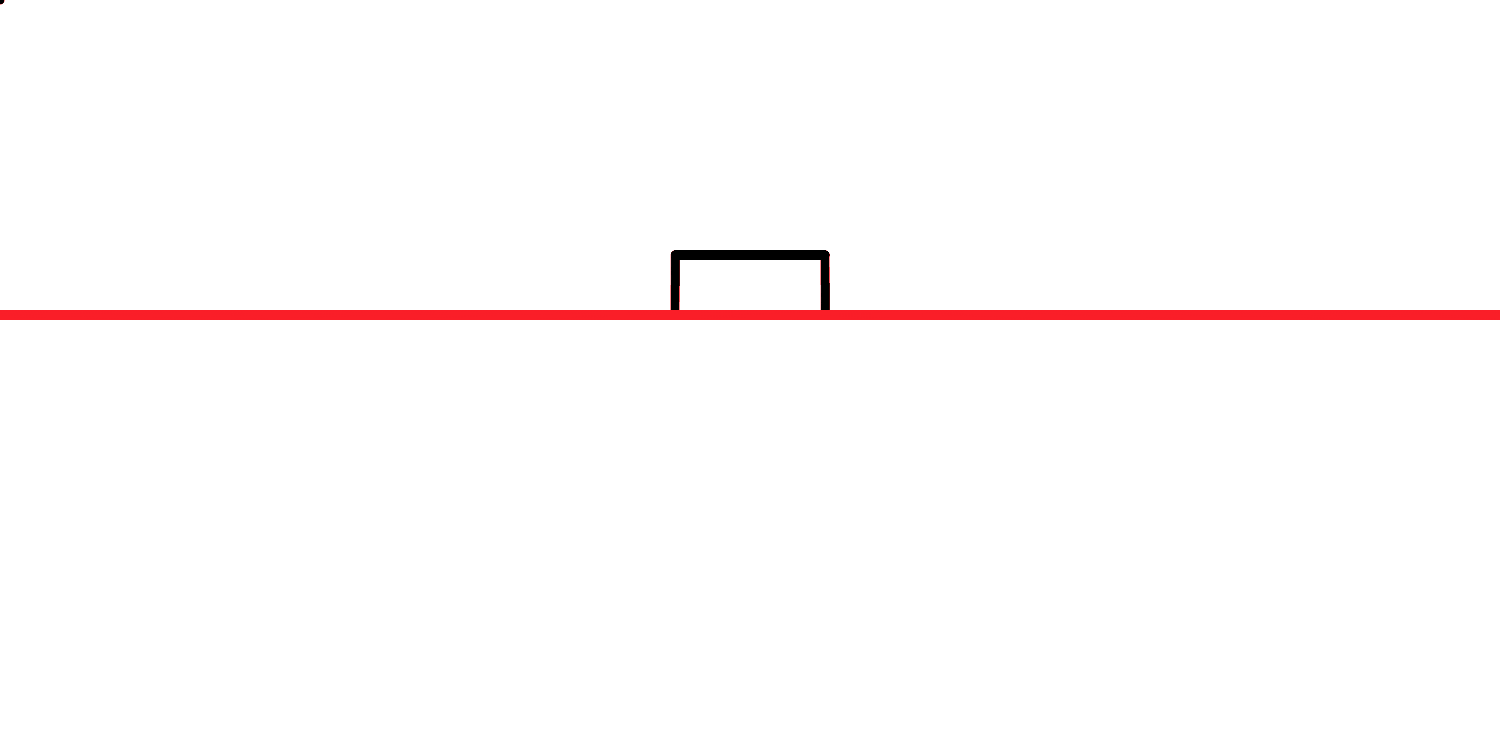TM

# Convolution

### What are we talking about?

A convolution function of two continuous functions $f(x)$ and $g(x)$ is defined as:

$(f \ast g)(t):=\int_{-\infty}^{\infty} f(\tau) g(t-\tau) d \tau$

You may ask: Hmm, okay, and what does that mean? Well, I did ask that myself, so here we are. In a nutshell, a convolution can be seen as a function that defines the overlap between the two functions $f(x)$ and $g(x)$, as $g(x)$ sweeps over $f(x)$. In this sense, $(f \ast g)(t)$ is kind of a mathematical “blend” of the two functions.

### Applications?

• image processing
• sound engineering
• quite a bit of physics
• and unsurprisingly a lot of heavy statistics, i.e. probability theory

Finally, here’s a little duck helping us with visualizing what’s going on when we convolve two uniform continuous functions.